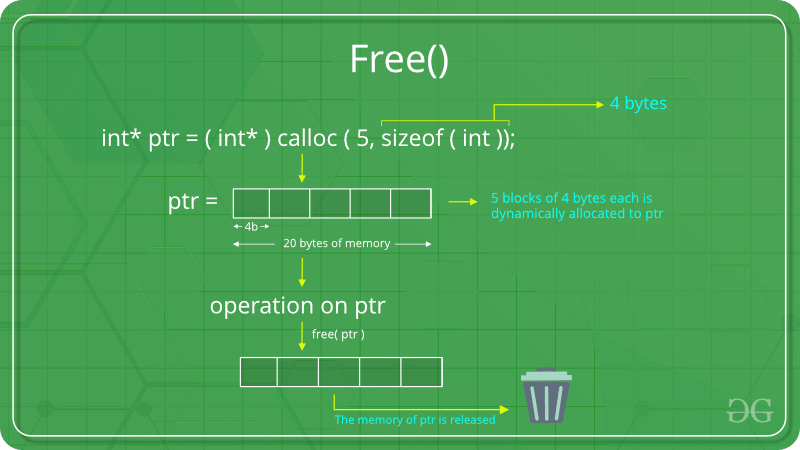Open In App

# free() Function in C Library With Examples

The free() function in C is used to free or deallocate the dynamically allocated memory and helps in reducing memory wastage. The C free() function cannot be used to free the statically allocated memory (e.g., local variables) or memory allocated on the stack. It can only be used to deallocate the heap memory previously allocated using malloc(), calloc() and realloc() functions.

The free() function is defined inside <stdlib.h> header file.C free() Function

## Syntax of free() Function in C

`void free(void *ptr);`

### Parameters

• ptr is the pointer to the memory block that needs to be freed or deallocated.

### Return Value

• The free() function does not return any value.

## Examples of free()

### Example 1:

The following C program illustrates the use of the calloc() function to allocate memory dynamically and  free() function to release that memory.

## C

 `// C program to demonstrate use of``// free() function using calloc()``#include ``#include ``int` `main()``{` `    ``// This pointer ptr will hold the``    ``// base address of the block created``    ``int``* ptr;``    ``int` `n = 5;` `    ``// Get the number of elements for the array``    ``printf``(``"Enter number of Elements: %d\n"``, n);` `    ``scanf``(``"%d"``, &n);` `    ``// Dynamically allocate memory using calloc()``    ``ptr = (``int``*)``calloc``(n, ``sizeof``(``int``));` `    ``// Check if the memory has been successfully``    ``// allocated by calloc() or not``    ``if` `(ptr == NULL) {``        ``printf``(``"Memory not allocated \n"``);``        ``exit``(0);``    ``}``    ``// Memory has been Successfully allocated using calloc()``    ``printf``(``"Successfully allocated the memory using "``           ``"calloc(). \n"``);` `    ``// Free the memory``    ``free``(ptr);` `    ``printf``(``"Calloc Memory Successfully freed."``);` `    ``return` `0;``}`

Output

```Enter number of Elements: 5
Successfully allocated the memory using calloc().
Calloc Memory Successfully freed.```

### Example 2:

The following C program illustrates the use of the malloc() function to allocate memory dynamically and  free() function to release that memory.

## C

 `// C program to demonstrate use of``// free() function using malloc()``#include ``#include ` `int` `main()``{` `    ``// This pointer ptr will hold the``    ``// base address of the block created``    ``int``* ptr;``    ``int` `n = 5;` `    ``// Get the number of elements for the array``    ``printf``(``"Enter number of Elements: %d\n"``, n);` `    ``scanf``(``"%d"``, &n);` `    ``// Dynamically allocate memory using malloc()``    ``ptr = (``int``*)``malloc``(n * ``sizeof``(``int``));` `    ``// Check if the memory has been successfully``    ``// allocated by malloc() or not``    ``if` `(ptr == NULL) {``        ``printf``(``"Memory not allocated \n"``);``        ``exit``(0);``    ``}``    ``// Memory has been Successfully allocated using malloc()``    ``printf``(``"Successfully allocated the memory using "``           ``"malloc(). \n"``);` `    ``// Free the memory``    ``free``(ptr);` `    ``printf``(``"Malloc Memory Successfully freed."``);` `    ``return` `0;``}`

Output

```Enter number of Elements: 5
Successfully allocated the memory using malloc().
Malloc Memory Successfully freed.```